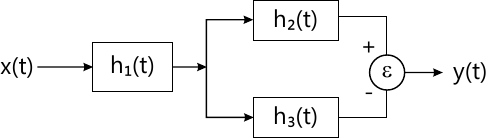MORE IN Signals & Systems
Total marks: --
Total time: --
INSTRUCTIONS
(1) Assume appropriate data and state your reasons
(2) Marks are given to the right of every question
(3) Draw neat diagrams wherever necessary

Solve any one question from Q1 and Q2
1 (a) Find odd and even components of following signals.
i) x(t) = 3-2t2+6t3+9t4.
ii) x[n]=u[n].
4 M
1 (b) Determine whether the following signals are periodic or aperiodic. If periodic find the fundamental period.
i) x(t)=3 sin (4πt)+7 cos (3πt).
ii) x[n]=cos(2n).
4 M
1 (c) Find the response of the LTI system for i/p $$x(t) = rest \left ( \dfrac {t}{2} \right ).$$ If the system is described by impulse response. h(t)=δ(t+1)-2δ(t)+δ(t-1).
4 M

2 (a) Sketch the following signals: $i) \ x(t)=u (t) + u(t-2)+ u(t-4)-3 u(t-6) \\ ii) \ x(t) = \sum^{10}_{k=-10} \delta (t-2k)$
4 M
2 (b) Classify whether the following system are:
a) Causal/Non-causal.
b) Stable/unstable.
i) h(t)=e2t u(-t)
ii) h[n]=δ[n]+δ[n-2]-2δ[n-3].
4 M
2 (c) Find the overall impulse response of the system given below:
i) h1(t)=δ(t),
h2(t)=u(t).
h3(t)=u(t-2).4 M

Solve any one question from Q3 and Q4
3 (a) Find the Fourier Transform of following signals.
i) x(t) = sgn (t).
ii) x(t) = rect(t).
6 M
3 (b) Find the transfer function and impulse response of the system describe by following differential equation. $\dfrac {d^2}{dt^2}y(t)+5 \dfrac {d}{dt}y(t)+6y(t) = \dfrac {dx}{dt} (t)+ x(t).$
6 M

4 (a) Using differentiation in frequency domain property. Find Fourier transform of:
y(t)=tx(t)
where x(t) = e-at u(t).
6 M
4 (b) Find initial and final value of following: $i) \ x(S) = \dfrac {2}{s(s^2 +3s+5)} \\ ii) \ x(S) = \dfrac {1}{S^2}$
6 M

Solve any one question from Q5 and Q6
5 (a) Prove that auto-correlation and ES.D from a Fourier transform pair. Verify the same for x(t)=e-atu (t).
7 M
5 (b) Compute cross correlation between given two sequences: $x_1 [n] = \{1, 1, \underset{\uparrow}{2} , -1 \} \\ x_2 [n] = \{ 1, \underset{\uparrow}{2}, 3, 4 \}$ Using analytical or graphical methods only sketch the output sequence.
6 M

6 (a) Find auto-correlation, PSD and power of given signal.
x(t)=2 cos t +3 cos 3t+5 sin 4 t.
7 M
6 (b) State and describe the properties of energy spectral density (ESD).
6 M

Solve any one question from Q7 and Q8
7 (a) PDF of a random variable 'X' is given as Fx(x)=e-x for x≥0.
Find:
i) Mean E[x].
ii) Mean square E[x2].
iii) Variance
iv) Std. deviation.
7 M
7 (b) Explain Gaussian probability model with respect to its density and distribution function.
6 M

8 (a) A random variable X is defined by the CDF. $F_x (x)= \left\{\begin{matrix} 0 & x<0 \\\ \dfrac {1}{2}x & 0 \le x \le 1 \\ k &x \ge 1 \end{matrix}\right.$ i) Find value of K.
ii) Find and sketch PDF.
iii) P(x>2).
7 M
8 (b) State and explain properties of PDF.
6 M

More question papers from Signals & Systems